,
Message sent from: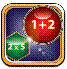Mathtopia Practise all 4 operations - first few levels are free.Math Sumo Practise maths skills against an opponent until one of you is pushed off the screen.Operation Math Work as a secret agent to destroy evil Dr Odd using basic maths facts.Number Run For younger children. Chase Baron von Count and develop an understanding of early number skills.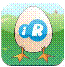Eggs on Legs Crack eggs by using calculation skills.Math Workout Answer 50 maths questions against the clock.Multiple Wipeout Pop multiples of numbers - good for learning tables.Motion Math Zoom Practise a variety of maths skills including decimals and negative numbers.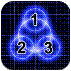Glow Burst Burst numbers in order. Fast paced and includes negative numbers.Fishy Numbers Mental addition.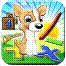Math Puppy Calculation games.Mathemagics Mental maths challenges to build up speed in calculation.Number Bonds Pro Practise number bonds. A similar Times Table Pro is also available.Maths v Zombies Protect the house from the zombies by answering maths questions which get increasingly challenging.Mathmateer Maths game with a space theme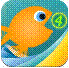Hungry Fish Simple addition game with correct answers making the fish grow.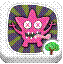Math Monsters Find the hidden monsters by solving calculations.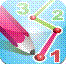Dot to Dot Numbers and Letters For younger children. Touch numbers in the correct order. . .

X